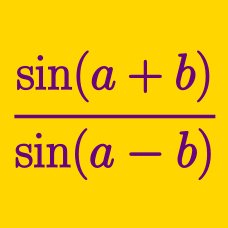Geometry

# Sine - Sum and Difference Formulas

Which of the following is equal to

$\sin 56 ^ \circ \cos 17 ^ \circ - \cos 56 ^ \circ \sin 17 ^ \circ ?$

$$\sin 43 ^ \circ \cos 82 ^ \circ + \cos 43 ^ \circ \sin 82 ^ \circ = \sin N ^\circ$$, where $$0 \leq N \leq 90$$. What is the value of $$N$$?

Which of the following is equal to

$\sin 29 ^ \circ \cos 31 ^ \circ + \cos 29 ^ \circ \sin 31 ^ \circ ?$

Using the Sine sum formula, what is the value of

$\sin 75 ^ \circ ?$

$$\sin 21 ^ \circ \cos 37 ^ \circ + \cos 21 ^ \circ \sin 37 ^ \circ = \sin N ^\circ$$, where $$0 \leq N \leq 90$$. What is the value of $$N$$?

×